# DATE Function – Create Date in Excel, VBA, Google Sheets

This tutorial demonstrates how to use the Excel DATE Function in Excel to create a date.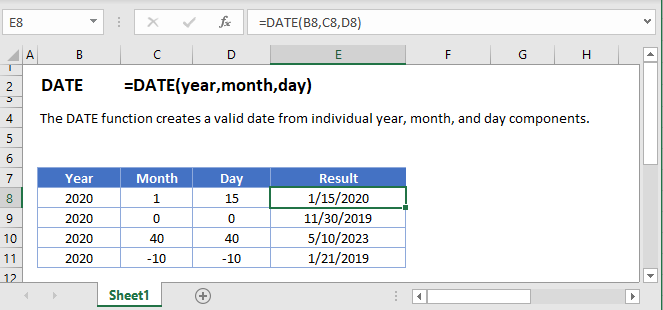## DATE Function Overview

The DATE Function Returns a date from year, month, and day.

To use the DATE Excel Worksheet Function, Select cell and Type: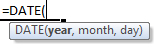(Notice how the formula input appear)
DATE Function syntax and Inputs:

year – A year greater than 1900 (1904 for Macs). Example: 2010.

month – The corresponding number of the month you wish to use (1-12). The month number can also be negative or greater than 12. Example: 2.

day – The day of the month you wish to use (1-31). The day number can also be negative or greater than 31. Example: 18.

## DATE Examples

The DATE Function is used to create dates by entering a year, month, and day: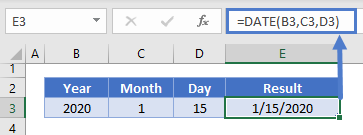Day and Month values can be zero: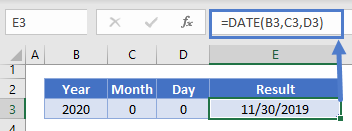or negative: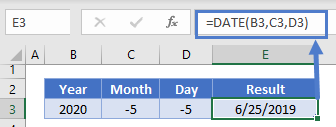### DATE – DAY, MONTH, YEAR Functions

Often the Date Function is used along with DAY, MONTH, or YEAR Functions to calculate a date based on another date.

This example will return the first day of the year based on a given date: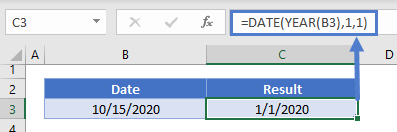### Date Serial Numbers

The DATE Function will return a serial number representing a date: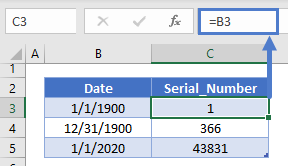The DATE Function works exactly the same in Google Sheets as in Excel: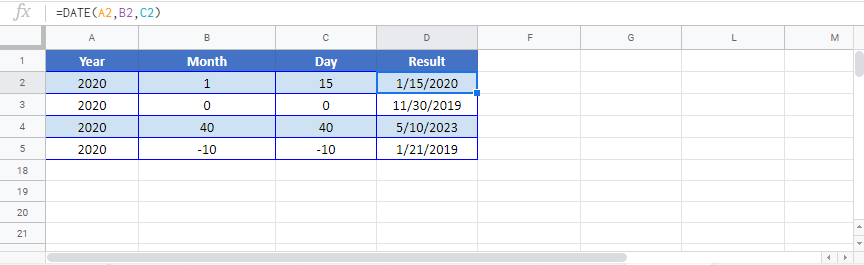Use the DATE Function to create a date serial number. Just enter numbers corresponding to the month, day, and year. You can actually enter months greater than 12 or less than 1 and Days greater than 31 and less than 1. Entering month=13 will set the date to January of the following year. Month=0 will set the date to December of the previous year.

Examples:
`=date(2016,0,0) returns 11/30/2015.`
From this starting point you can add or subtract months and days.

`=date(2016,13,1) returns 1/1/2017`

`=date(2016,-5,1) returns 7/1/2015`

The result of the DAY Function may appear as a serial number. Change the cell Number Formatting to Short Date to display the serial number as a date:## DATE Examples in VBA

You can also use the DATE function in VBA. Type:
`application.worksheetfunction.date(year,month,day)`
For the function arguments (year, etc.), you can either enter them directly into the function, or define variables to use instead.